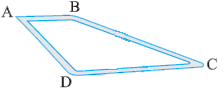# Ex 2.5 Q7 Fractions and Decimals Solution- NCERT Maths class 7

Go back to  'Ex.2.5'

## Question

Dinesh went from place $$A$$ to place $$B$$ and from there to place $$C$$. $$A$$ is $$7.5\,\rm{km}$$ from $$B$$ and $$B$$ is $$12.7\,\rm{km}$$ from $$C$$. Ayub went from place $$A$$ to place $$D$$ and from there to place $$C$$. $$D$$ is $$9.3\,\rm{km}$$ from $$A$$ and $$C$$ is $$11.8\,\rm{km}$$ from $$D$$.Who travelled more and how much ?Video Solution
Fractions And Decimals
Ex 2.5 | Question 7

## Text Solution

What is known?

Distance between points. Dinesh went from place $$A$$ to $$B$$ and then $$B$$ to $$C$$. Ayub went from place $$A$$ to $$D$$ then $$D$$ to $$C$$.

What is unknown?

Who travelled more and how much.

Reasoning:

Calculate by all the distance travelled by Dinesh to how much he travelled then calculate distance travel by Ayub.

Steps:

GivenDistance travelled by Dinesh from $$A$$ to $$B = 7. 5\,\rm{km}$$

And from place $$B$$ to place $$C = 12.7 \,\rm{km}$$

$$\therefore$$ Total distance travelled by Dinesh

\begin{align} &= AB+ BC\\&= 7.5\,\rm{km} + 12.7\,\rm{km}\\&= 20.2 \,\rm{km}\end{align}

Distance travelled by Ayub from place $$A$$ to place $$D = 9.3 \,\rm{km}$$

And from place $$D$$ to place $$C = 11.8\,\rm{km}$$

$$\therefore$$ Total distance travelled by Ayub

\begin{align}&= 9.3\,\rm{km} + 11.8 \,\rm{km}\\&= 21.1 \,\rm{km}\end{align}

On comparing the total distance travelled by Dinesh and Ayub, we get

$$21.1\,\rm{km}$$$$>$$ $$20.2\,\rm{km}$$

Distance travelled by Ayub > Distance travelled by Dinesh

$$\therefore$$ Ayub covered more distance by Dinesh is

\begin{align}&= 21.1 -20.2\\&= 0.9 \,\rm{km}\\&= 0.9 × 1000 \,\rm{m}\\&= 900\,\rm{m}\end{align}

Learn from the best math teachers and top your exams

• Live one on one classroom and doubt clearing
• Practice worksheets in and after class for conceptual clarity
• Personalized curriculum to keep up with school